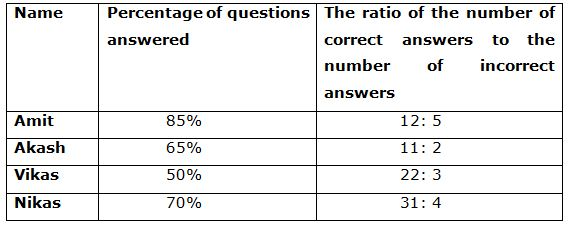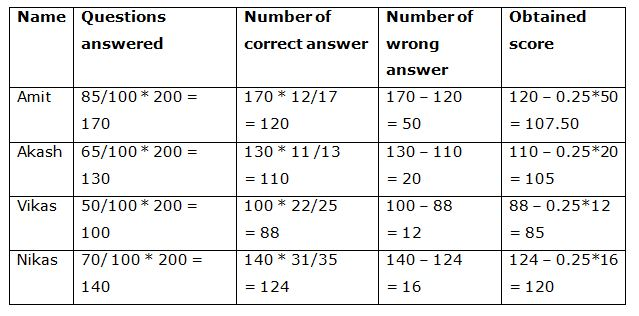# IBPS PO Prelims Quantitative Aptitude Questions 2019 (Day-10)

Dear Aspirants, Our IBPS Guide team is providing new series of Quantitative Aptitude Questions for IBPS PO Prelims 2019 so the aspirants can practice it on a daily basis. These questions are framed by our skilled experts after understanding your needs thoroughly. Aspirants can practice these new series questions daily to familiarize with the exact exam pattern and make your preparation effective.

Check here for IBPS PO Prelims Mock Test 2019

##### Check here for IBPS PO  Mock Test 2019
[WpProQuiz 7053]

Directions (1 – 5): Find out the wrong number in the following number series.

1) 1333, 1731, 2201, 2749, 3378

a) 1333

b) 2201

c) 1731

d) 3378

e) 2749

2) 16, 40, 72, 106, 148, 196

a) 16

b) 72

c) 148

d) 106

e) 196

3) 7, 8, 18, 57, 232, 1160

a) 57

b) 232

c) 7

d) 1160

e) 18

4) 71, 80, 96, 121, 159, 206

a) 159

b) 96

c) 121

d) 80

e) 206

5) 321, 316, 324, 309, 329, 306

a) 324

b) 326

c) 303

d) 321

e) 309

Directions (6 – 10): Study the following information carefully and answer the questions given below.

Four students appeared in an examination having 200 questions. The table given below shows the percentage of questions answered out of the total number of questions by each of the four students. It also shows the ratio of the number of correct answers to the number of incorrect answers.

Note: For each correct answer the student is given mark and for every incorrect answer 0.25 marks are deducted. No marks are deducted for un – attempted questions.6) Number of incorrect answers marked by Nikas is what percentage More/less than the number of incorrect answers marked by Akash?

a) 20% More

b) 30% More

c) 40% Less

d) 20% Less

e) None of these

7) What is the difference between the marks scored by Vikas and Akash?

a) 20

b) 30

c) 35

d) 15

e) 25

8) What is the ratio of correct answers marked by Amit to the wrong answers marked by Nikas?

a) 2: 15

b) 3: 16

c) 15: 2

d) 3: 2

e) 4: 5

9) Who scored the 2nd highest marks?

a) Amit

b) Nikas

c) Vikas

d) Akash and Amit

e) Akash

10) What is the score obtained by Amit?

a) 110.25

b) 120

c) 125.75

d) 107.50

e) 105.75

Direction (1-5) :

11³ + 2 = 1333

12³ + 3 = 1731

13³ + 4 = 2201

14³ + 5 = 2749

15³ + 6 = 3381

16 + 24 = 40

40 + 30 = 70

70 + 36 = 106

106 + 42 = 148

148 + 48 = 196

7 * 1 + 1 = 8

8 * 2 + 2 = 18

18 * 3 + 3 = 57

57 * 4 + 4 = 232

232 * 5 + 5 = 1165

71 + 3² = 80

80 + 4² = 96

96 + 5² = 121

121 + 6² = 157

157 + 7² = 206

321 – 5 = 316

316 + 8 = 324

324 – 12 = 312

309 + 17 = 329

329 – 23 = 306

Directions (6 – 10):Number of incorrect answers marked by Nikas = 16

the number of incorrect answers marked by Akash = 20

Required percentage = (20-16) / 20 * 100

= 20 % Less

Marks scored by Vikas = 85

Marks scored by Akash = 105

Difference = 20

Correct answers marked by Amit = 120

Wrong answers marked by Nikas = 16

Ratio = 120/16 = 15: 2

Mark of,

Amit = 107.5

Akash = 105

Vikas = 85

Nikas = 120

Amit has 2nd highest mark.